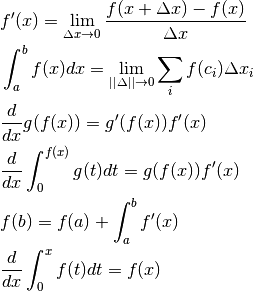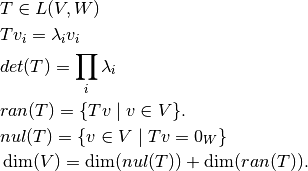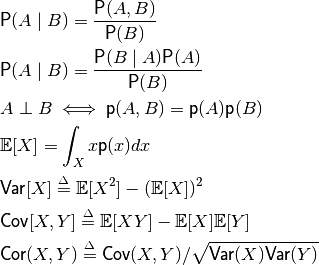or

# Mathematics¶

## Calculus¶

#### CalculusShow/Hide Equations## Linear Algebra¶

#### Linear AlgebraShow/Hide Equations## Probability Theory¶

#### Probability TheoryShow/Hide Equations